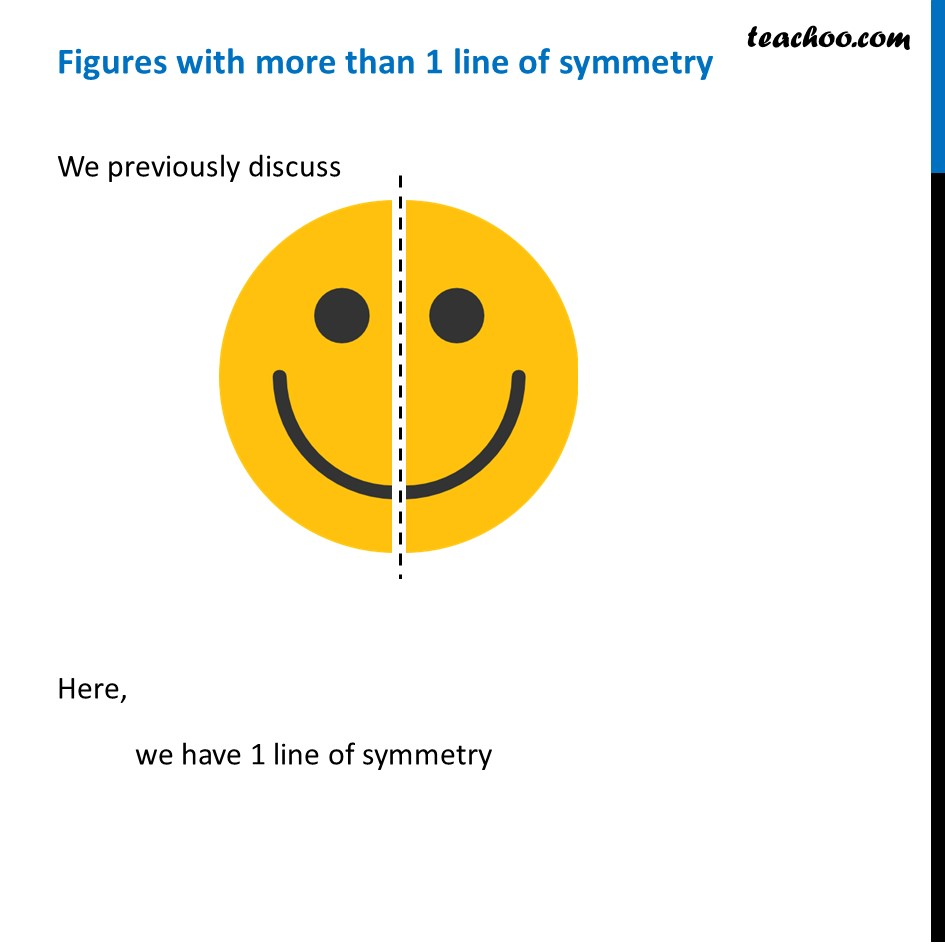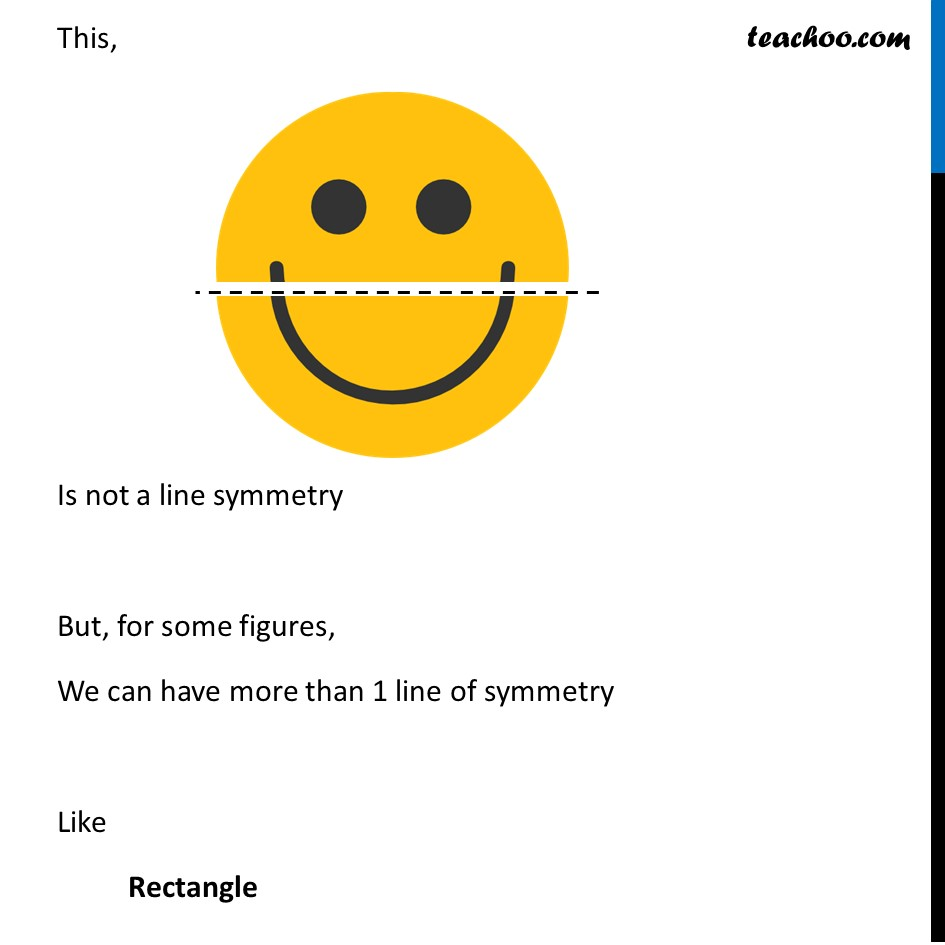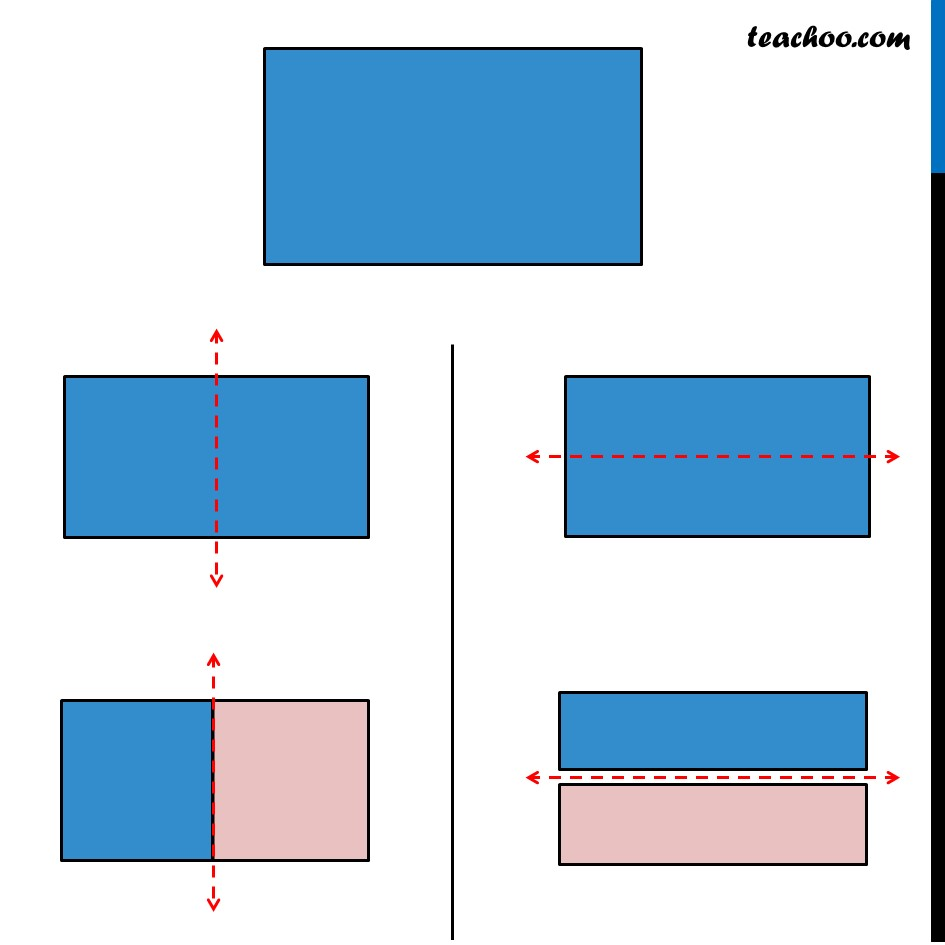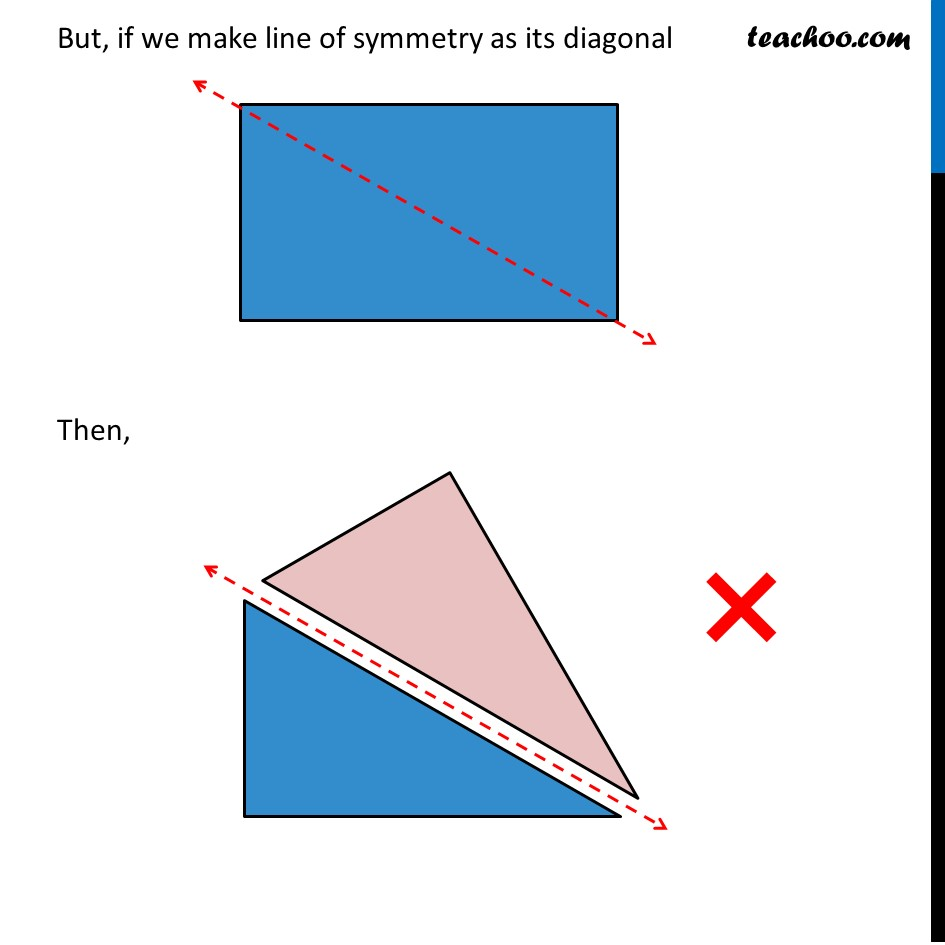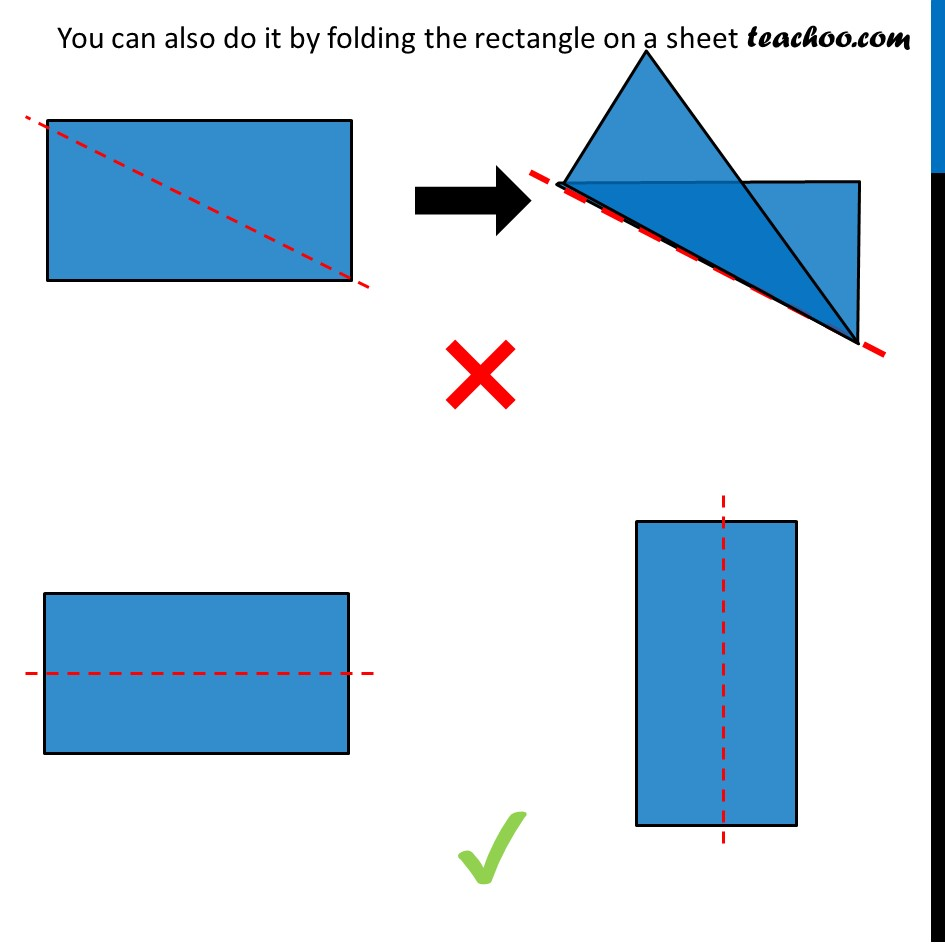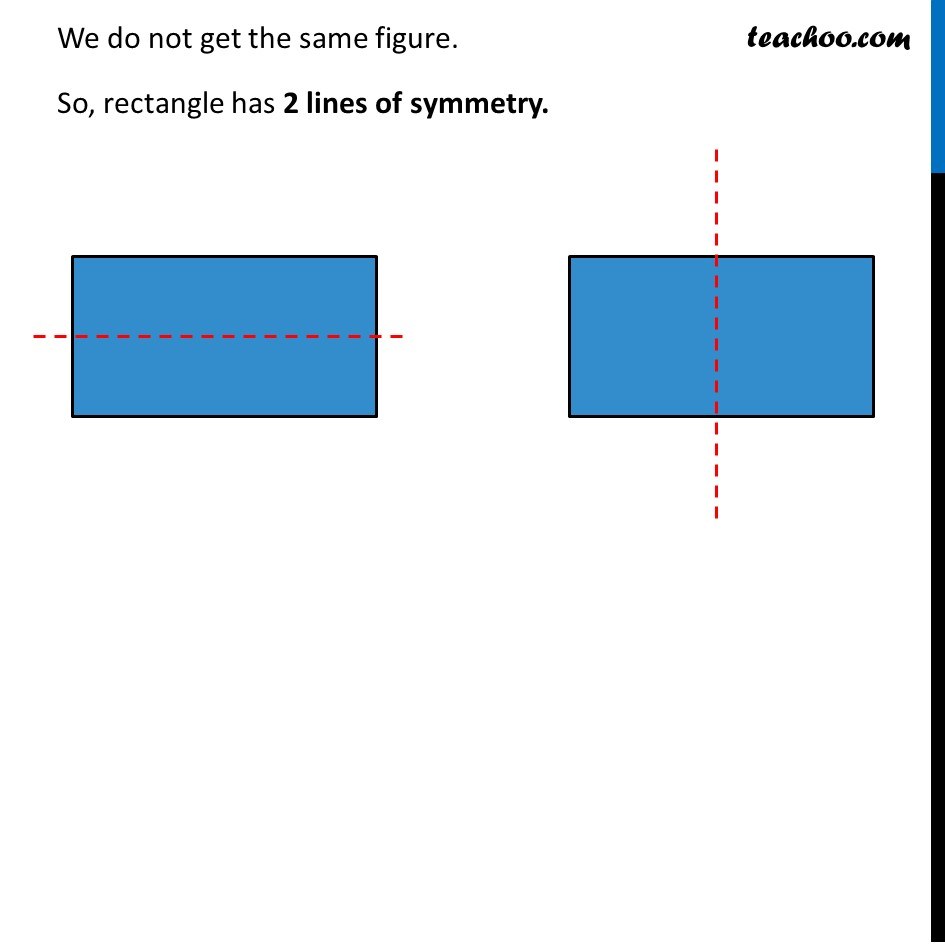1. Chapter 13 Class 6 Symmetry
2. Concept wise
3. Figures with more than one lines of symmetry

Transcript

Figures with more than 1 line of symmetryWe previously discuss Here, we have 1 line of symmetry This, Is not a line symmetry But, for some figures, We can have more than 1 line of symmetry Like Rectangle But, if we make line of symmetry as its diagonal Then, You can also do it by folding the rectangle on a sheet We do not get the same figure. So, rectangle has 2 lines of symmetry. Figures with more than 1 line of symmetryWe previously discuss Here, we have 1 line of symmetry This, Is not a line symmetry But, for some figures, We can have more than 1 line of symmetry Like Rectangle But, if we make line of symmetry as its diagonal Then, You can also do it by folding the rectangle on a sheet We do not get the same figure. So, rectangle has 2 lines of symmetry.

Figures with more than one lines of symmetry

Chapter 13 Class 6 Symmetry
Concept wise

About the AuthorDavneet Singh
Davneet Singh is a graduate from Indian Institute of Technology, Kanpur. He has been teaching from the past 10 years. He provides courses for Maths and Science at Teachoo.